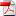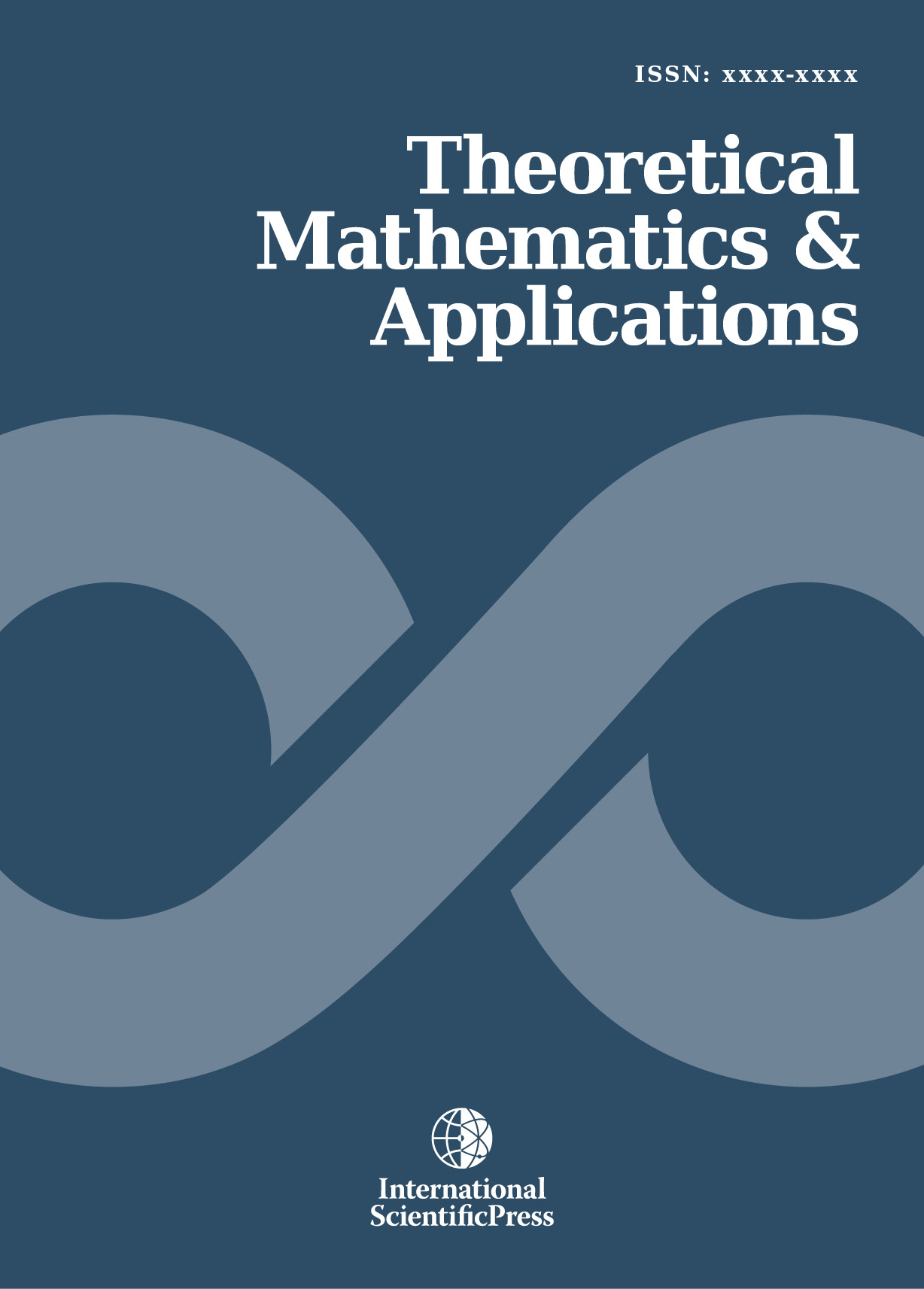# Theoretical Mathematics & Applications

#### The n2 + 1 Fermat and Mersenne prime numbers conjectures are resolved

•[ Download ]
• Abstract

In 1912 in Cambridge, the fourth problem mentioned by Landau in the Fifth Congress of Mathematicians was the conjecture that there are infinitely many primes p of the form p = n2 + 1. In 1640, the French mathematician Fermat conjectured that all numbers Fn = 2^2^n+ 1 were prime. Today, this conjecture has become that there are no Fermat prime numbers greater than F4 = 2^2^4 +1 = 65537. In 1644, the French Minim Friar Marin Mersenne conjectured that the function fn = 2n - 1 generates prime numbers only for n = 2, 3, 5,

7, 13, 17, 19, 31, 67, 127 and 257. Today, we know that this is wrong for 67 and 257 and that 48 Mersenne prime numbers exist. The conjecture is now that there are infinitely many Mersenne prime numbers. As of 2015, these three conjectures are unresolved. The main contribution of this paper is to introduce a new approach to these questions. The key idea of this new approach is that these problems can be solved by a system made of an appropriate test and a congruence with fixed modulus, both dedicated to each kind of number.ISSN: 1792-9687 (Print)
1792-9709 (Online)